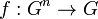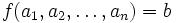# Multiary quasigroup

A multiary quasigroup or polyadic quasigroup is a$n$-ary quasigroup for some$n \ge 2$. Note that the$n = 2$ case corresponds to the usual notion of quasigroup.
A$n$-ary quasigroup is defined as a set$G$ with a$n$-ary operation, i.e., a map$f: G^n \to G$ such that the equation$f(a_1,a_2,\dots,a_n) = b$ has a unique solution with any$a_i$ as the unknown and all other quantities (i.e., all the other <mah>a_j[/itex]s and$b$) known.